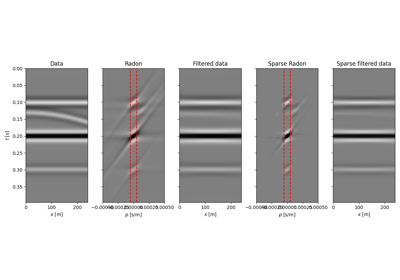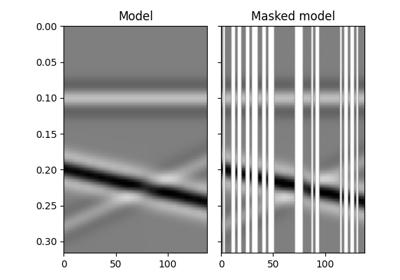# pylops.utils.seismicevents.linear2d#

pylops.utils.seismicevents.linear2d(x, t, v, t0, theta, amp, wav)[source]#

Linear 2D events

Create 2d linear events given propagation velocity, intercept time, angle, and amplitude of each event

Parameters
xnumpy.ndarray

space axis

tnumpy.ndarray

time axis

vfloat

propagation velocity

t0tuple or float

intercept time at $$x=0$$ of each linear event

thetatuple or float

angle (in degrees) of each linear event

amptuple or float

amplitude of each linear event

wavnumpy.ndarray

wavelet to be applied to data

Returns
dnumpy.ndarray

data without wavelet of size $$[n_x \times n_t]$$

dwavnumpy.ndarray

data with wavelet of size $$[n_x \times n_t]$$

Notes

Each event is created using the following relation:

$t_i(x) = t_{0,i} + p_{x,i} x$

where $$p_{x,i}=\sin( \theta_i)/v$$

## Examples using pylops.utils.seismicevents.linear2d#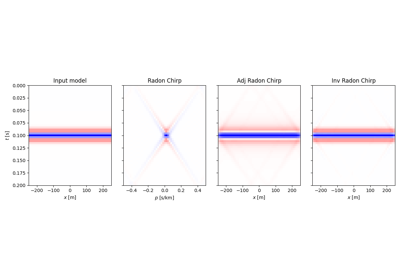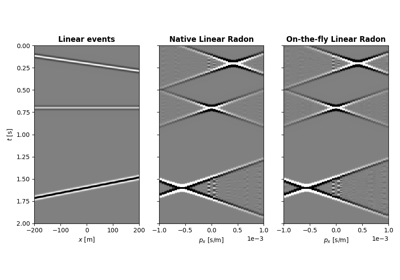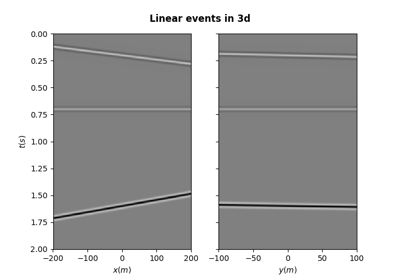Synthetic seismic

Synthetic seismic